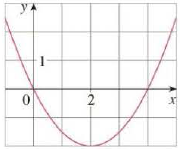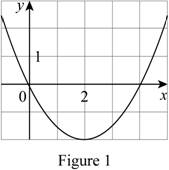Chapter 10.5, Problem 10E

Chapter
Section
Textbook Problem

# Find an equation of the parabola. Then find the focus and directrix.10.To determine

To Find: The equation of the parabola, focus and the directrix by using figure (1).Explanation

Given:

By referring the figure (1), we can find the value of vertex.  Then the vertex is (2,2).

Calculation:

By referring the figure (1) graph is open up upward in the direction of y, so the equation,

x2=4py (1)

And the focus of the parabola is to be (0,p).

Compute the value of the p in equation below.

(xh)2=4p(yk) (2)

Substitute the value 2 for h and 2 for k in the equation (1).

(xh)2=4p(yk)(x2)2=4p(y(2))

(x2)2=4p(y+2) (3)

Substitute the value 0 for x and 0 for y in the equation (3).

(02)2=4p(0+2)4=8pp=12

Compute the focus of the parabola:

Focus=(h,k+p)

Substitute the value 12 for p2 for h and 2 for k

### Still sussing out bartleby?

Check out a sample textbook solution.

See a sample solution

#### The Solution to Your Study Problems

Bartleby provides explanations to thousands of textbook problems written by our experts, many with advanced degrees!

Get Started

#### Evaluate the integral. 02(45t334t2+25t)dt

Single Variable Calculus: Early Transcendentals, Volume I

#### Find for defined implicity by .

Study Guide for Stewart's Multivariable Calculus, 8th

#### For y=12gt2 (where g is a constant), dydt=. a) 14g2 b) 12g c) gt d) gt2

Study Guide for Stewart's Single Variable Calculus: Early Transcendentals, 8th

#### What is the goal of a single-case experimental research design?

Research Methods for the Behavioral Sciences (MindTap Course List)